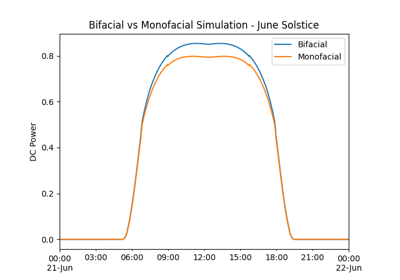# pvlib.pvsystem.pvwatts_dc#

pvlib.pvsystem.pvwatts_dc(g_poa_effective, temp_cell, pdc0, gamma_pdc, temp_ref=25.0)[source]#

Implements NREL’s PVWatts DC power model. The PVWatts DC model 1 is:

$P_{dc} = \frac{G_{poa eff}}{1000} P_{dc0} ( 1 + \gamma_{pdc} (T_{cell} - T_{ref}))$

Note that the pdc0 is also used as a symbol in pvlib.inverter.pvwatts(). pdc0 in this function refers to the DC power of the modules at reference conditions. pdc0 in pvlib.inverter.pvwatts() refers to the DC power input limit of the inverter.

Parameters
• g_poa_effective (numeric) – Irradiance transmitted to the PV cells. To be fully consistent with PVWatts, the user must have already applied angle of incidence losses, but not soiling, spectral, etc. [W/m^2]

• temp_cell (numeric) – Cell temperature [C].

• pdc0 (numeric) – Power of the modules at 1000 W/m^2 and cell reference temperature. [W]

• gamma_pdc (numeric) – The temperature coefficient of power. Typically -0.002 to -0.005 per degree C. [1/C]

• temp_ref (numeric, default 25.0) – Cell reference temperature. PVWatts defines it to be 25 C and is included here for flexibility. [C]

Returns

pdc (numeric) – DC power.

References

1

## Examples using pvlib.pvsystem.pvwatts_dc#